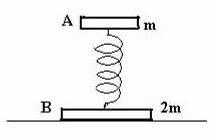## Monday, January 01, 2007

### Additional Multiple Choice Questions on Simple Harmonic Motion

The following post is in continuation of the post ‘Multiple Choice Questions on Simple Harmonic Motion’, dated 19th October 2006:
Let us consider the following MCQ which appeared in Kerala Engineering Entrance 2005 question paper:
A particle starts SHM from its mean position. Its amplitude is ‘a’ and total energy is ‘E’. At one instant its kinetic energy is 3E/4. Its displacement at that instant is
(a) a/√2 (b) a/2 (c) a/√(3/2) (d) a/√3 (e) a
The equation of the simple harmonic motion is y = A sinωt with usual notations. The total energy is (½) m ω2a2 and the kinetic energy is (½)m ω2(a2 –y2).
Therefore we have (½) m ω2a2 = E and
(½)m ω2( a2 –y2) = 3E/4.
Dividing the second equation by the first, 1 – y2/a2 = ¾, from which y = a/2.
The following question will be interesting to you:Two discs A and B of masses m and 2m respectively are connected by a light spring and placed on a table (Fig.) so that the disc B is on the table and the disc A is resting on the spring. If the frequency of oscillation of the spring on slightly depressing and releasing the disc A, is ‘f’, the frequency of oscillation on suddenly removing the table is (a) f/2 (b) f/√2 (c) f (d) √(2/3) f (e) √(3/2) f
When disc B is resting on the table, disc A functions as the oscillating mass in a spring-mass system and the period of oscillation, as usual, is given by T = 2π√(m/k) where ‘k’ is the spring constant. The frequency of oscillation is f = (1/2π)√(k/m).
When the table is removed, the system falls freely and both discs are free to oscillate. The reduced mass (μ) of the vibrating system is given by μ = m×2m/(m+2m) = (2/3)m.
The frequency of vibration in this case is obtained by replacing ‘m’ in the above expression for frequency by (2/3)m so that the new frequency is (1/2π)√[k/(2/3)m] = (1/2π)√(k/m) ×√(3/2) = f ×√(3/2) [Option (e)].
Now, consider the following question:
The kinetic energy and potential energy of a simple harmonic motion (of amplitude ‘A’ ) will be equal when the displacement is
(a) A/√2 (b) A√2 (c) A/2 (d) A/3 (e) A/√3
Equating the kinetic energy to the potential energy, we have ½ m ω2( A2 –y2) = ½ m ω2y2, from which ( A2 –y2) = y2. Therefore, y = A/√2.
Consider the following MCQ:
Two light springs of force constants k1 and k2, loaded with equal masses undergo vertical oscillations. If the maximum velocities of the masses are the same, the ratio of the amplitudes of oscillations is
(a) k1 /k2 (b) 1 (c) k1 2 /k2 2 (d) k2 /k1 (e) √(k2 /k1 )
If A1 and A2 are the amplitudes of oscillations of the first and the second system respectively and ω1 and ω2 their angular frequencies, we have ω1 A1 = ω2 A2 , since the maximum velocities are equal. Therefore, A1 /A2 = ω21 .
But ω = √(k/m) for linear simple harmonic motion so that A1 /A2 = √(k2 /m2 )/ √(k1 /m1 ) = √(k2 /k1 ) since the masses are equal.
[Even if you don’t remember the relation, ω = √(k/m), you can work out this since the maximum potential energy (and maximum kinetic energy) of an oscillating spring is ½kA2 . Since the maximum velocities are equal (as specified in the question), the maximum kinetic energies are equal so that we have, ½k1 A1 2 =½k2 A2 2 , from which A1 /A2 = √(k2 /k1 )].